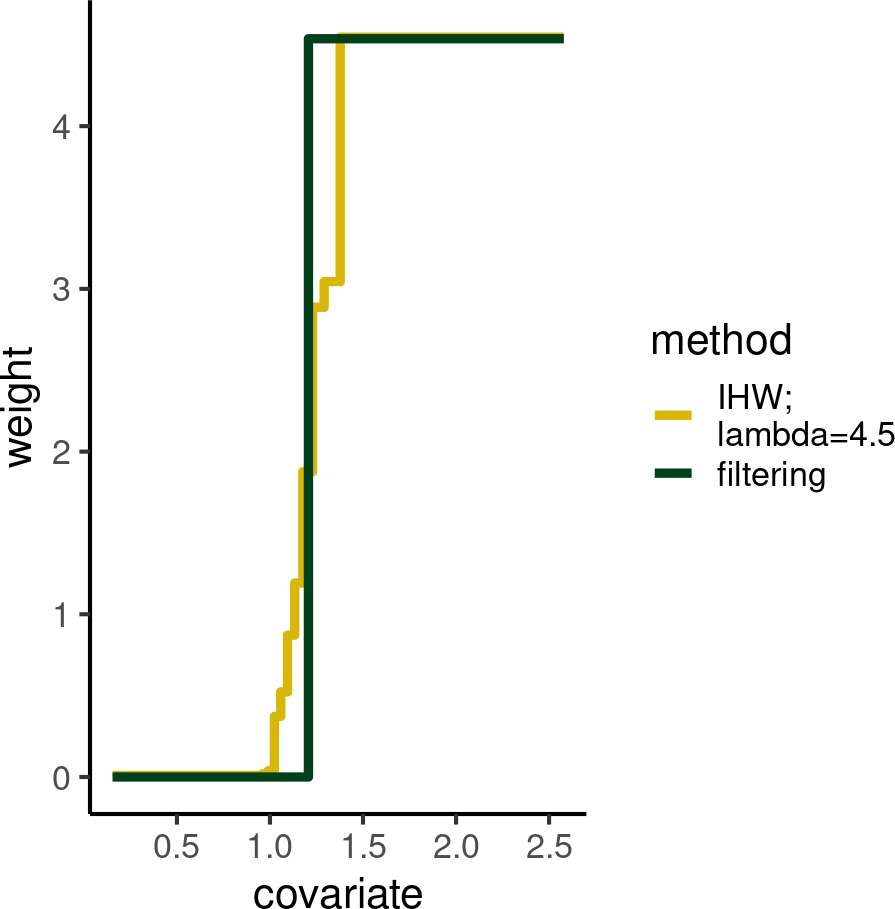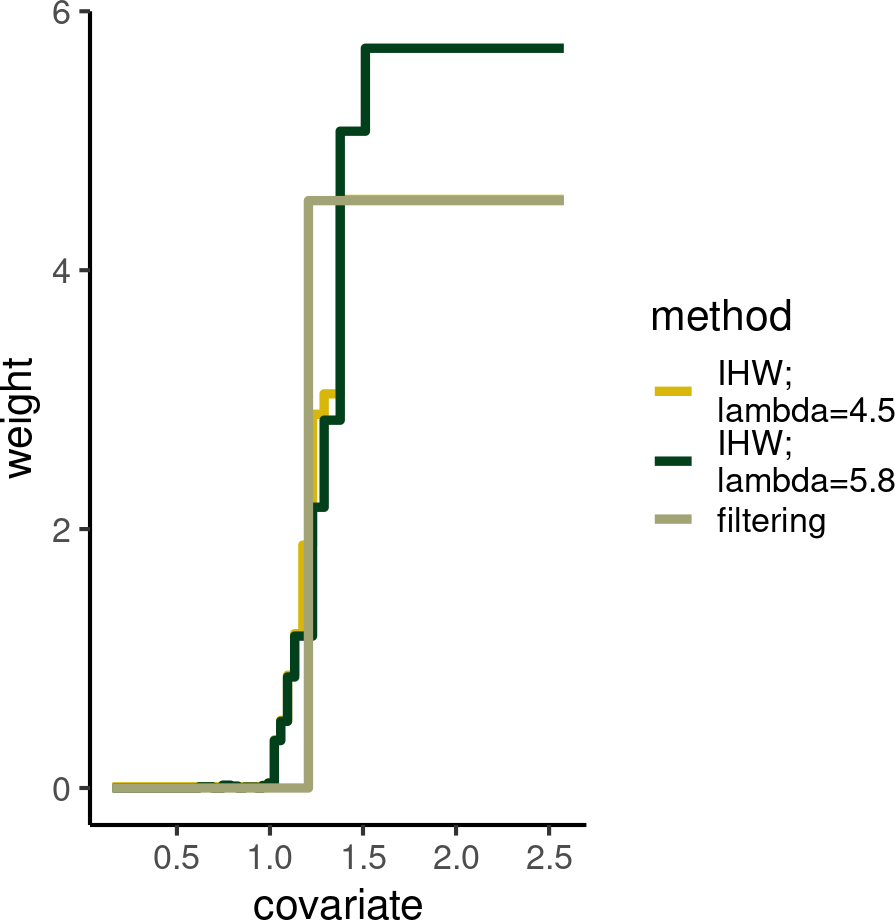In this vignette we show that weights can behave very similarly to an independent filtering threshold:

library("IHW")
library("IHWpaper")
library("ggplot2")
library("dplyr")
library("wesanderson")

First we generate the simulated data and then use (data-driven greedy) independent filtering. We convert the binary threshold cut-off into weights.

sim <- du_ttest_sim(80000, 0.9, 2, seed=1)
ddhf_res <- ddhf(sim$pvalue, sim$filterstat, .1)
ws <- ifelse(sim$filterst <= ddhf_res$cutoff_value, 0, 1)
ws <- ws/sum(ws)*length(sim$pvalue) The induced weight function has total regularization equal to the following: total_regularization_lambda <- max(ws)-min(ws) total_regularization_lambda ##  4.537205 We now apply IHW based on the above total regularization penalization parameter. This makes the comparison more directly applicable. ihw_res <- ihw(sim$pvalue, sim$filterstat, .1, lambdas = total_regularization_lambda, nfolds=1L, nbins=20) ## Using only 1 fold! Only use this if you want to learn the weights, but NEVER for testing! The plot below compares the binary threshold with the IHW threshold, which is a bit smoother: df <- rbind( data.frame(covariate = sim$filterstat, weight= ws, method="filtering"),
data.frame(covariate = sim$filterstat, weight= weights(ihw_res, levels_only=FALSE), method=paste0("IHW; \nlambda=",format(total_regularization_lambda,digits=2)))) weights_filter_plot <- ggplot(df, aes(x=covariate, y=weight, col=method)) + geom_step(size=1.65)+ scale_colour_manual(values=wes_palette("Cavalcanti1")[c(1,2)]) + theme_classic(16) weights_filter_plotpdf("smoothed_threshold.pdf", width=5, height=5) weights_filter_plot dev.off() But weights can be even more flexible if we allow the total regularization parameter to vary. Here we use 5fold CV along an equally spaced grid of lambdas to find the best total variation regularization using IHW: ihw_res2 <- ihw(sim$pvalue, sim$filterstat, .1, lambdas=seq(0,10,length=20), nfolds=1L, nfolds_internal = 5L, nbins=20, quiet=TRUE) The returned regularization parameter is the following: regularization_term(ihw_res2) ##  5.789474 Now we plot everything: df <- rbind(df, data.frame(covariate = sim$filterstat, weight= weights(ihw_res2, levels_only=FALSE),
method=paste0("IHW; \nlambda=",
format(regularization_term(ihw_res2) ,digits=2))))

weights_filter_plot <- ggplot(df, aes(x=covariate, y=weight, col=method)) + geom_step(size=1.65)+
scale_colour_manual(values=wes_palette("Cavalcanti1")[c(1,2,3)]) +
theme_classic(16)

weights_filter_plotNotice that all 3 types of weights still fullfill the necessary budget condition:

group_by(df, method) %>% summarize(mean_weight = mean(weight))
## # A tibble: 3 × 2
##   method              mean_weight
##   <chr>                     <dbl>
## 1 "IHW; \nlambda=4.5"           1
## 2 "IHW; \nlambda=5.8"           1
## 3 "filtering"                   1